## Recommended Posts

ben voila ds des boukin de prog il parle de notation hexadecimal avec 0x eske qq1 pourai m expliker celo et comment le faqire corespondre a un chiffre

merci

##### Share on other sites

pour convertir un nombre hexa en décimale, rien de plus simple. Prends ton nombre en hexa et sépare le en chiffres (0,1,2,3,4,5,6,7,8,9,a,b,c,d,e,f) et en partant de gauche multiplie le par des puissances de 16 successives.

ex : 0x 123c= (16^0)x12 + (16^1)x3 + (16^2)x2 + (16^3)x4.

Je te laisses faire le calcul. Sinon pour pas te prendre la tête, le plus simple est de faire un prog.

##### Share on other sites

Je vient de retrouver un vieux prog qui me servait bien. Si le nombre commence par 0 (on suppose qu'il y a un x derrière 0x) alors il converti hexa-> décimal. sinon, il convertit décimal -> hexa.

#include<stdio.h>

int main(void)

{

char buff;

long number;

long i;

char hexa;

while((buff=getchar())!=45)

{

if(buff==48)

{

getchar();

number=0;

while((buff=getchar())!='n')

{

if(buff>64)

number=16*number+buff-55;

else

number=16*number+buff-48;

}

i=10;

while(i<number)

i*=10;

while(i=i/10)

{

putchar(number%i+48);

number/=10;

}

putchar('n');

}

else

{

number=buff-48;

while((buff=getchar())>47 && buff<58)

number=10*number+buff-48;

i=0;

while(number)

{

hexa=number%16;

if(hexa>9)

hexa[i++]+=55;

else

hexa[i++]+=48;

number/=16;

}

putchar(48);

putchar(120);

while(i)

{

putchar(hexa[--i]);

}

putchar(10);

}

}

}

##### Share on other sites

Pour précision, on rajoute généralement le '0' ou '0x' devant une notation hexa pour ne pas la confondre avec une étiquette en assembleur par exemple.

##### Share on other sites

pour convertir un nombre hexa en décimale, rien de plus simple. Prends ton nombre en hexa et sépare le en chiffres (0,1,2,3,4,5,6,7,8,9,a,b,c,d,e,f) et en partant de gauche multiplie le par des puissances de 16 successives.

ex : 0x 123c= (16^0)x12 + (16^1)x3 + (16^2)x2 + (16^3)x4.

Je te laisses faire le calcul. Sinon pour pas te prendre la tête, le plus simple est de faire un prog.

g pa compris ton exemple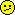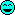peut tu reexpliker plz je suis un peu con je sais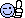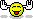##### Share on other sites

pour convertir un nombre hexa en décimale, rien de plus simple. Prends ton nombre en hexa et sépare le en chiffres (0,1,2,3,4,5,6,7,8,9,a,b,c,d,e,f) et en partant de gauche multiplie le par des puissances de 16 successives.

ex : 0x 123c= (16^0)x12 + (16^1)x3 + (16^2)x2 + (16^3)x4.

Je te laisses faire le calcul. Sinon pour pas te prendre la tête, le plus simple est de faire un prog.

heuu, petite erreur, il fallait lire

0x 123c= (16^0)x12 + (16^1)x3 + (16^2)x2 + (16^3)x1

Une autre solution est de séparer les chiffres en partant de la droite et de les multiplier par 16. Avec le même exemple, ça donne :

0x c = c*1= 12*1

0x 3c = ((c*1)+3)*16 = ((12*1)+3)*16

0x 23c = ((((c*1)+3)*16)+2)*16

0x 123c = ((((((c*1)+3)*16)+2)*16)+1)*16

##### Share on other sites

pour convertir un nombre hexa en décimale, rien de plus simple. Prends ton nombre en hexa et sépare le en chiffres (0,1,2,3,4,5,6,7,8,9,a,b,c,d,e,f) et en partant de gauche multiplie le par des puissances de 16 successives.

ex : 0x 123c= (16^0)x12 + (16^1)x3 + (16^2)x2 + (16^3)x4.

Je te laisses faire le calcul. Sinon pour pas te prendre la tête, le plus simple est de faire un prog.

heuu, petite erreur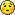, il fallait lire

0x 123c= (16^0)x12 + (16^1)x3 + (16^2)x2 + (16^3)x1

Une autre solution est de séparer les chiffres en partant de la droite et de les multiplier par 16. Avec le même exemple, ça donne :

0x c = c*1= 12*1

0x 3c = ((c*1)+3)*16 = ((12*1)+3)*16

0x 23c = ((((c*1)+3)*16)+2)*16

0x 123c = ((((((c*1)+3)*16)+2)*16)+1)*16

en fait ds le 1ere exemple c en partan de droite pa de gauche (voir ta premiere reponse )

##### Share on other sites

Chuuuuuut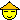Oui, en fait ça fait deux erreurs. Faut pas le dire trop fort..

##### Share on other sites

ChuuuuuutOui, en fait ça fait deux erreurs. Faut pas le dire trop fort..

bon je me tait mtn ok ?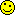en fait c ke j av un doute donc bon je preferai l eclairer ms bon faut pa t ecuser c deja sympa d avoir repondu (a plusieur reprise)

PS:l erreur est humaine.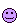##### Share on other sites

ChuuuuuutOui, en fait ça fait deux erreurs. Faut pas le dire trop fort..

bon je me tait mtn ok ? :8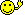en fait c ke j av un doute donc bon je preferai l eclairer ms bon faut pa t ecuser c deja sympa d avoir repondu (a plusieur reprise)

PS:l erreur est humaine.Je me suis pas excusé, j'ai humblement reconnu mes erreurs.(bon joueur quand même).

Errare humanum est, perseverare diabolicum.

Sinon, ravi de t'avoir aidé.

##### Share on other sites

une solution hyper rapide pour calculer : utiliser la calculatrice de windows

il suffit de passer en mode scientifique

par défaut, la calculatrice est placée en 'dec' --> décimal , dès que tu saisis la valeur, tu sélectionnes 'hex' et hop tu as la valeur hexadécimal

et tu peux l'avoir aussi en octal

chouette comme joujou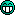##### Share on other sites

Salut!

la notation "0x.." indique que dans ton programme tu utilises une valeur en hexadecimal.

Par exemple

en binaire => 10101010

Perso,j'utilise cette notation essentiellement pour le langage C.

Pour l'assembleur,on notera "h'AA"

##### Share on other sites

Pour l'assembleur,on notera "h'AA"

Non, pour l'assembleur, en tout ces pour les asm Intel, c'est :

```
5860h           ;si t'as que des chiffres

0AAFh          ;si tu commences par une lettre, pour pas confondre avec les etiquettes

```

Tu peux meme utiliser 'RADIX 16' pour changer la base de travail par defaut, ici changee en base 16 (hexa).

##### Share on other sites

Ben excuse moi mais c'est ce que j'utilise (la notation h'AA) pour programmer les micro-controleurs Pic16f8xx et ça fonctionne à merveille

##### Share on other sites

J'ai bien dit sur les CPU Intel, je pensais que tu parlais d'ASM Intel ou AMD, et non pas de PIC, alors prenons mon post comme un complément de réponse :-)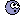##### Share on other sites

prenons mon post comme un complément de réponse :-)Vi vi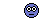De toutes façons, je crois que les jeux d'instructions sont differents (entre cpu et micro-controleur) c'est pour ça qu'il y'a eu confusion ...à confirmer bien sûre (je n'ai jamais etudié la structure des cpu!!!)

Jeremy > Au fait, si tu veux faire des conversions hexa>bin ou hexa>déc ,etc... tu peux prendre une calculette (des fx4200 aux graph100).

Perso je n'utilise pas de calculette car ça embrouille au niveau du binaire (sens de lecture/ecriture).Mais c'est un moyen comme un autre!

##### Share on other sites

Sinon il y a aussi le printf pour afficher en hexa :o)

`printf("Valeur decimal : %d et hexa %Xn",mavaleur,mavaleur);`

##### Share on other sites

Ben ton printf sert à affiché le resultat!!!

Il manque la partie la plus importante de ton programme;La conversion!!!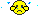non???

##### Share on other sites

Exact, le printf le fait tout seul. Mais il me semble que ce n'est pas ce qu'il voulait.

##### Share on other sites

Ben ton printf sert à affiché le resultat!!!

Il manque la partie la plus importante de ton programme;La conversion!!!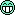non???

non nonTu peux mettre

`mavaleur = 12;`

ou bien

`mavaleur=0xD;`

c'est pareil...

Effectivement, ça ne répond pas à la question originelle... mais les options des Xprintf/Xscanf sont trop souvent mal connues ! Alors si j'peux ajouter mon grain d'sel;o)

##### Share on other sites

Alors si j'peux ajouter mon grain d'sel :8 ;o)

Oui n'hésite pas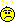Moi je galère un peu en C (ce n'est pas ma spécialité)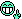##### Share on other sites

Je vient de retrouver un vieux prog qui me servait bien. Si le nombre commence par 0 (on suppose qu'il y a un x derrière 0x) alors il converti hexa-> décimal. sinon, il convertit décimal -> hexa.

#include<stdio.h>

int main(void)

{

char buff;

long number;

long i;

char hexa;

while((buff=getchar())!=45)

{

if(buff==48)

{

getchar();

number=0;

while((buff=getchar())!='n')

{

if(buff>64)

number=16*number+buff-55;

else

number=16*number+buff-48;

}

i=10;

while(i<number)

i*=10;

while(i=i/10)

{

putchar(number%i+48);

number/=10;

}

putchar('n');

}

else

{

number=buff-48;

while((buff=getchar())>47 && buff<58)

number=10*number+buff-48;

i=0;

while(number)

{

hexa=number%16;

if(hexa>9)

hexa[i++]+=55;

else

hexa[i++]+=48;

number/=16;

}

putchar(48);

putchar(120);

while(i)

{

putchar(hexa[--i]);

}

putchar(10);

}

}

}

Salut!

Je viens d'essayé ce programme mais je ne comprend pas car il ne fait que convertir le decimal en hexa et pas l'inverse!

##### Share on other sites

Salut!

Je viens d'essayé ce programme mais je ne comprend pas car il ne fait que convertir le decimal en hexa et pas l'inverse!

Tu peux essayer ça :

```
#include <stdio.h>

#include <stdlib.h>

int main(int argc,char * argv[])

{

char *endp;

char s;

long l;

if(gets(s))//on prend la premiere ligne entree

{

l = strtol(s, &endp, 0);//tentative de convertion

if (s != endp && *endp == '')//si tout s'est bien passe...

printf("Valeur en decimal = %ld, en hexa = %lXn", l,l);//...on affiche

else

return 1;//sinon on retourne en erreur

}

else

return 1;//erreur lors de la saisie

return 0;//OK

}```

Les hexas commencent bien sur par 0x... ou 0X...

##### Share on other sites

Arfff , j'arrive pas à le compiler (fonction declared implicit int (warning))

Merci quand même! en plus y'a des commentaires explicatifs ce que j'apréci beaucoup!##### Share on other sites

Je vient de retrouver un vieux prog qui me servait bien. Si le nombre commence par 0 (on suppose qu'il y a un x derrière 0x) alors il converti hexa-> décimal. sinon, il convertit décimal -> hexa.

#include<stdio.h>

int main(void)

{

char buff;

long number;

long i;

char hexa;

while((buff=getchar())!=45)

{

if(buff==48)

{

getchar();

number=0;

while((buff=getchar())!='n')

{

if(buff>64)

number=16*number+buff-55;

else

number=16*number+buff-48;

}

i=10;

while(i<number)

i*=10;

while(i=i/10)

{

putchar(number%i+48);

number/=10;

}

putchar('n');

}

else

{

number=buff-48;

while((buff=getchar())>47 && buff<58)

number=10*number+buff-48;

i=0;

while(number)

{

hexa=number%16;

if(hexa>9)

hexa[i++]+=55;

else

hexa[i++]+=48;

number/=16;

}

putchar(48);

putchar(120);

while(i)

{

putchar(hexa[--i]);

}

putchar(10);

}

}

}

Salut!

Je viens d'essayé ce programme mais je ne comprend pas car il ne fait que convertir le decimal en hexa et pas l'inverse!

Ton nombre décimal doit commencer par 0x (le nombre 0, pas la lettre O)

exemple d'entree :

0x54AE

84313

0xB55

sinon tu peut tester (j'ai pas encore compilé):

#include<stdio.h>

int main()

{

char buff;

long num;

while(1)

{

buff=getchar();

getchar();

if(buff=='h' || buff=='H')

{

scanf("%X",&num);

getchar();

printf("%ldn",num);

}

else

{

scanf("%ld",&num);

getchar();

printf("%Xn",num);

}

}

return 0;

}

exemple d'entree :

d

16513

h

0ab54d

etc..